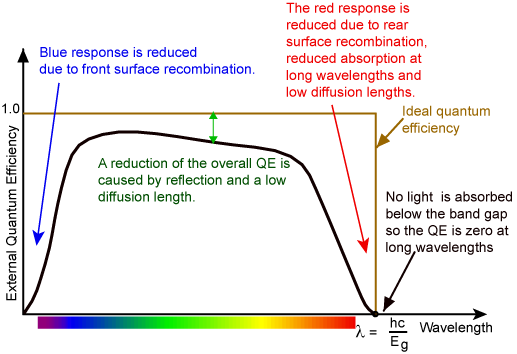# Quantum Efficiency

The "quantum efficiency" (Q.E.) is the ratio of the number of carriers collected by the solar cell to the number of photons of a given energy incident on the solar cell. The quantum efficiency may be given either as a function of wavelength or of energy. If all photons of a certain wavelength are absorbed and the resulting minority carriers are collected, then the quantum efficiency at that particular wavelength is unity. The quantum efficiency for photons with energy below the band gap is zero. A quantum efficiency curve for an ideal solar cell is shown below by the tan/gold square line.The quantum efficiency of a silicon solar cell. Quantum efficiency is usually not measured much below 350 nm as the power from the AM1.5 spectrum contained in such low wavelengths is low.

While quantum efficiency ideally has the square shape shown above, the quantum efficiency for most solar cells is reduced due to recombination effects. The same mechanisms which affect the collection probability also affect the quantum efficiency. For example, front surface passivation affects carriers generated near the surface, and since blue light is absorbed very close to the surface, high front surface recombination will affect the "blue" portion of the quantum efficiency. Similarly, green light is absorbed in the bulk of a solar cell and a low diffusion length will affect the collection probability from the solar cell bulk and reduce the quantum efficiency in the green portion of the spectrum. The quantum efficiency can be viewed as the collection probability due the generation profile of a single wavelength, integrated over the device thickness and normalized to the incident number of photons at that wavelength.

The "external" quantum efficiency of a silicon solar cell includes the effect of optical losses such as transmission and reflection. However, it is often useful to look at the quantum efficiency of the light left after the reflected and transmitted light has been lost. "Internal" quantum efficiency refers to the efficiency with which photons that are not reflected or transmitted out of the cell can generate collectable carriers. By measuring the reflection and transmission of a device, the external quantum efficiency curve can be corrected to obtain the internal quantum efficiency curve.

The animation below shows the effect on surface recombination and diffusion length on the internal quantum efficiency of a solar cell. The emitter thickness is 1 µm, the base thickness is 300 µm, the emitter diffusivity is 4 cm2s-1 and the base diffusivity is 27 cm2s-1. For base diffusion lengths greater than the device thickness of 300 µm the rear surface recombination velocity has a large effect on QE. For low diffusion lengths recombination at the rear surface has no effect.

X
1.010
X
1.01e6
X
1010000
X
1.01e6

Internal quantum efficiency. Lp is the emitter diffusion length (µm), Sp is the front surface recombination velocity (cm/s), Ln is the base diffusion length (µm), Sn is the rear surface recombination velocity (cm/s).